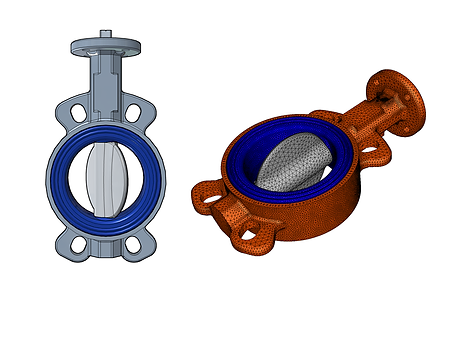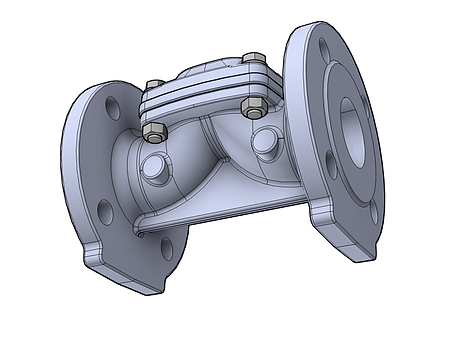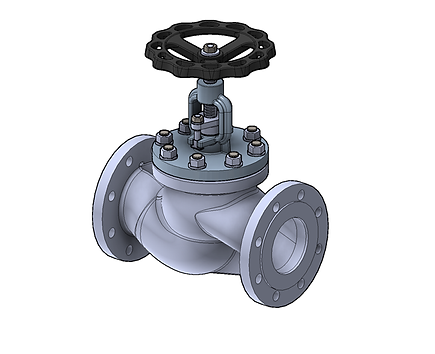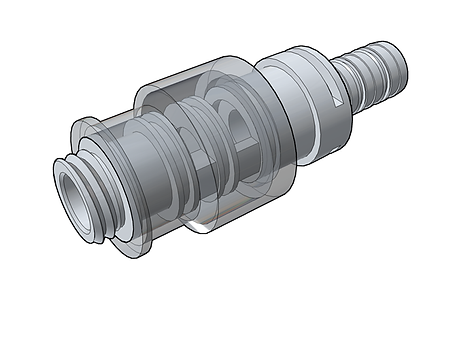## FLOW CONTROL

Projects/Flow  ControlButterfly Valve

In the project, the water flow in a butterfly valve was examined with CFD analysis, the valve flow capacity factor (Kv coefficient), the forces and moments acting on the damper were calculated.# .

Fire Hydrant (Above Ground)

In the project, the water flow in an above-ground fire hydrant was examined by CFD analysis, the hydrant flow capacity factor (Kv coefficient), the forces acting on the damper, the moments.  and from the hydrant  water flow  calculated.# .

Globe Valve

In the project, the water flow in a globe valve was examined with CFD analysis, the valve flow capacity factor (Kv coefficient), the forces and moments acting on the damper were calculated.# .

Control Valve

In the project, the water flow in a control valve was examined by CFD analysis, the valve flow capacity factor (Kv coefficient) and  The forces acting on the diaphragm were calculated.# .

Y-Type Control Valve

In the project, the water flow in a Y-type control valve was examined by CFD analysis, and the valve flow capacity factor (Kv coefficient) and the forces acting on the diaphragm were calculated.# .

Globe Valve

In the project, the water flow in a globe valve was examined with CFD analysis, the valve flow capacity factor (Kv coefficient), the forces and moments acting on the damper were calculated.# .

Plastic Control Valve

In the project, the water flow in a plastic control valve was examined by CFD analysis, the valve flow capacity factor (Kv coefficient) and  The forces acting on the diaphragm were calculated.# .

Butterfly Valve

In the project, the water flow in a butterfly valve was examined with CFD analysis, the valve flow capacity factor (Kv coefficient), the forces and moments acting on the damper were calculated.# .

Balance Valve

In the project, the water flow in a balancing valve was examined by CFD analysis, the valve flow capacity factor (Kv coefficient), the forces acting on the flap,  moments and   Depending on the pressure, the water flow rates that will pass through the valve are calculated.# .

Fire Hydrant (Above Ground)

In the project, the water flow in an above-ground fire hydrant was examined by CFD analysis, the hydrant flow capacity factor (Kv coefficient), the forces acting on the damper, the moments.  and from the hydrant  water flow  calculated.# .

Fire Hydrant (Underground)

In the project, the water flow in an underground fire hydrant was examined by CFD analysis, the hydrant flow capacity factor (Kv coefficient), the forces acting on the damper, the moments.  and from the hydrant  water flow  calculated.# .

Dynamic Balance Valve

In the project, the water flow in a dynamic balancing valve was examined by CFD analysis, the valve flow capacity factor (Kv coefficient), the forces acting on the flap,  moments and   Depending on the pressure, the water flow rates that will pass through the valve are calculated.# .

Pressure Relief Valve

In the project, the gas flow in a pressure relief valve was examined by CFD analysis,  Depending on the pressure, the gas flow rate that can be discharged from the valve was calculated, and the discharge capacity was desired with the design improvements.  level has been raised.# .

Air Relief Valve

In the project, the air flow in an air release valve (suction cup) was examined by CFD analysis,  The air flow that can be discharged from the valve is calculated.# .

Check Valve

In the project, the water flow in a check valve was examined by CFD analysis, the flow capacity factor (Kv coefficient) depending on the damper angle, the forces acting on the damper,  moments and   flow rates were calculated.